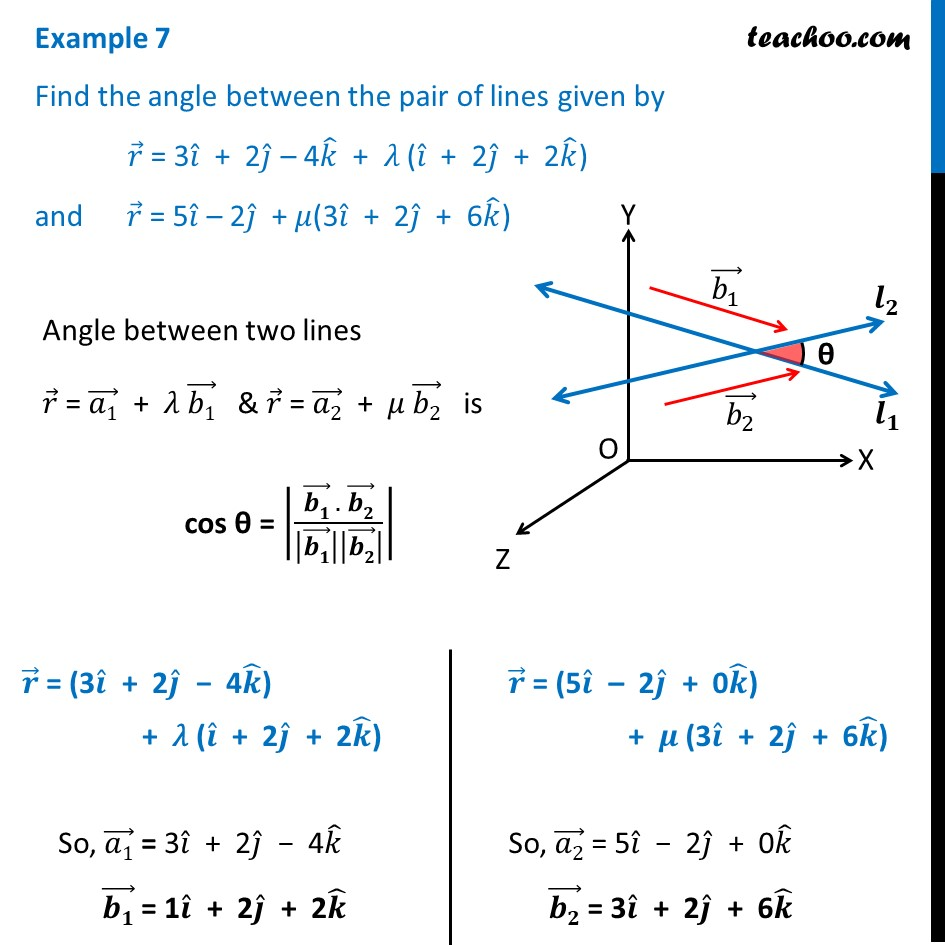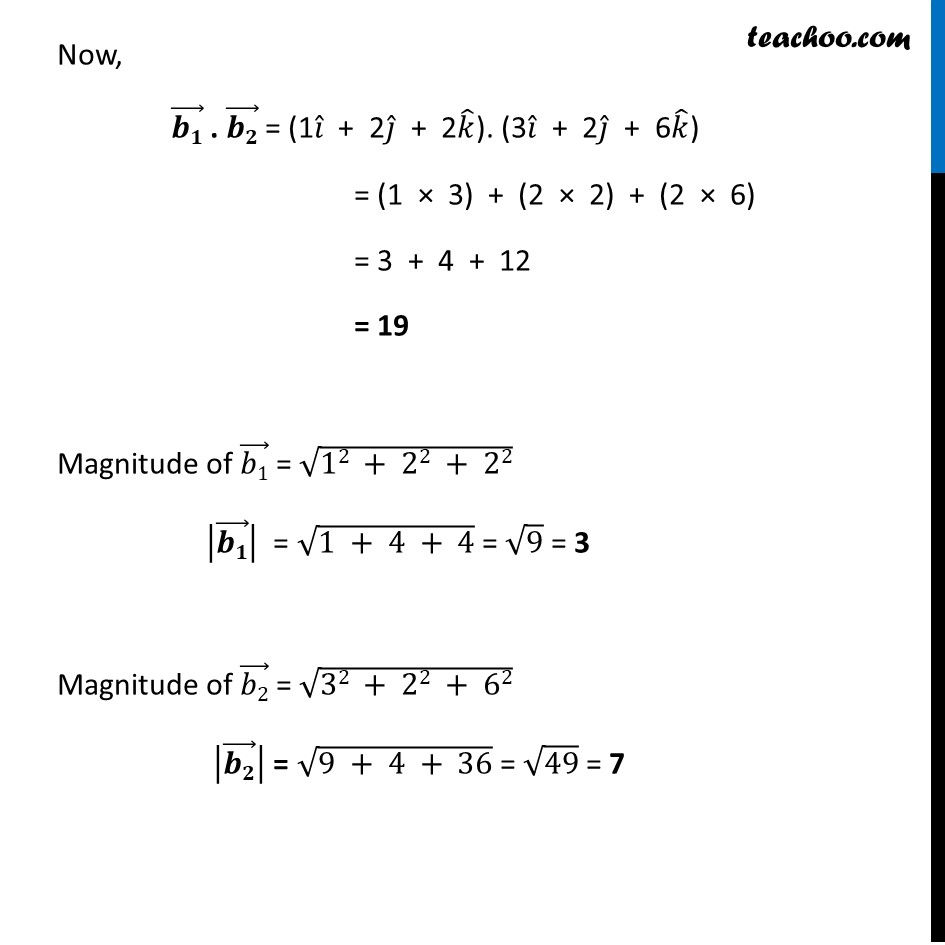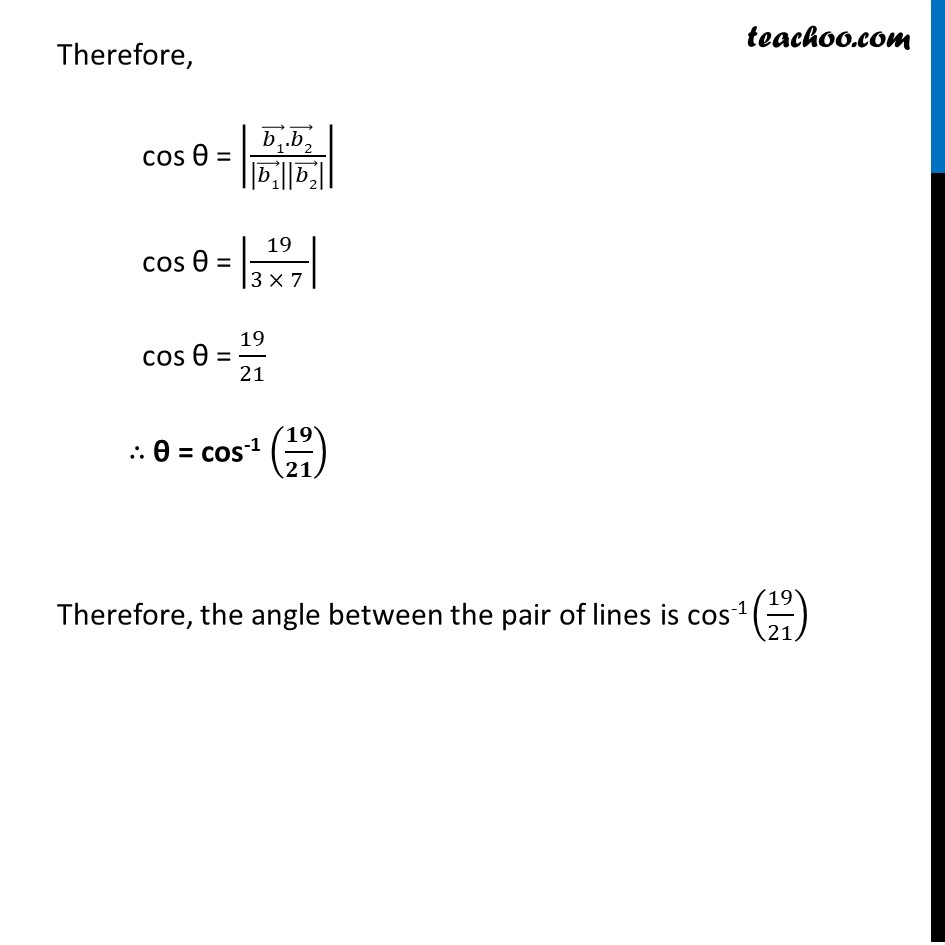Examples

Chapter 11 Class 12 Three Dimensional Geometry
Serial order wiseLearn in your speed, with individual attention - Teachoo Maths 1-on-1 Class

### Transcript

Example 7 Find the angle between the pair of lines given by 𝑟 ⃗ = 3𝑖 ̂ + 2𝑗 ̂ – 4𝑘 ̂ + 𝜆 (𝑖 ̂ + 2𝑗 ̂ + 2𝑘 ̂) and 𝑟 ⃗ = 5𝑖 ̂ – 2𝑗 ̂ + 𝜇(3𝑖 ̂ + 2𝑗 ̂ + 6𝑘 ̂)Angle between two lines 𝑟 ⃗ = (𝑎1) ⃗ + 𝜆 (𝑏1) ⃗ & 𝑟 ⃗ = (𝑎2) ⃗ + 𝜇 (𝑏2) ⃗ is cos θ = |((𝒃𝟏) ⃗ . (𝒃𝟐) ⃗)/|(𝒃𝟏) ⃗ ||(𝒃𝟐) ⃗ | | 𝒓 ⃗ = (3𝒊 ̂ + 2𝒋 ̂ − 4𝒌 ̂) + 𝜆 (𝒊 ̂ + 2𝒋 ̂ + 2𝒌 ̂) So, (𝑎1) ⃗ = 3𝑖 ̂ + 2𝑗 ̂ − 4𝑘 ̂ (𝒃𝟏) ⃗ = 1𝒊 ̂ + 2𝒋 ̂ + 2𝒌 ̂ 𝒓 ⃗ = (5𝒊 ̂ – 2𝒋 ̂ + 0𝒌 ̂) + 𝝁 (3𝒊 ̂ + 2𝒋 ̂ + 6𝒌 ̂) So, (𝑎2) ⃗ = 5𝑖 ̂ − 2𝑗 ̂ + 0𝑘 ̂ (𝒃𝟐) ⃗ = 3𝒊 ̂ + 2𝒋 ̂ + 6𝒌 ̂ Now, (𝒃𝟏) ⃗ . (𝒃𝟐) ⃗ = (1𝑖 ̂ + 2𝑗 ̂ + 2𝑘 ̂). (3𝑖 ̂ + 2𝑗 ̂ + 6𝑘 ̂) = (1 × 3) + (2 × 2) + (2 × 6) = 3 + 4 + 12 = 19 Magnitude of (𝑏1) ⃗ = √(12 + 22 + 22) |(𝒃𝟏) ⃗ | = √(1 + 4 + 4) = √9 = 3 Magnitude of (𝑏2) ⃗ = √(32 + 22 + 62) |(𝒃𝟐) ⃗ | = √(9 + 4 + 36) = √49 = 7 Therefore, cos θ = |((𝑏1) ⃗.(𝑏2) ⃗)/|(𝑏1) ⃗ ||(𝑏2) ⃗ | | cos θ = |19/(3 × 7 )| cos θ = 19/21 ∴ θ = cos-1 (𝟏𝟗/𝟐𝟏) Therefore, the angle between the pair of lines is cos-1 (19/21)